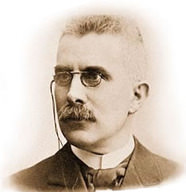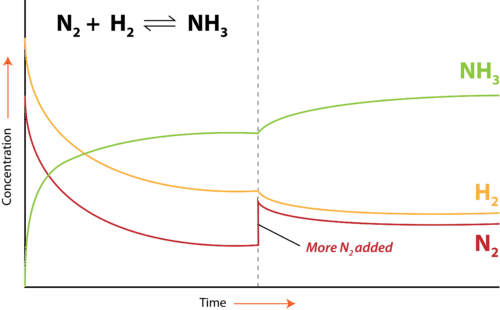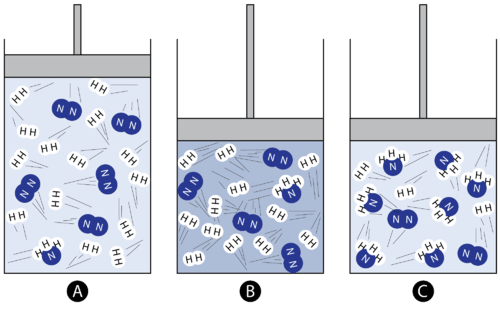# 5.7: Le Chatelier's Principle

•• Anonymous
• LibreTexts
$$\newcommand{\vecs}{\overset { \rightharpoonup} {\mathbf{#1}} }$$ $$\newcommand{\vecd}{\overset{-\!-\!\rightharpoonup}{\vphantom{a}\smash {#1}}}$$$$\newcommand{\id}{\mathrm{id}}$$ $$\newcommand{\Span}{\mathrm{span}}$$ $$\newcommand{\kernel}{\mathrm{null}\,}$$ $$\newcommand{\range}{\mathrm{range}\,}$$ $$\newcommand{\RealPart}{\mathrm{Re}}$$ $$\newcommand{\ImaginaryPart}{\mathrm{Im}}$$ $$\newcommand{\Argument}{\mathrm{Arg}}$$ $$\newcommand{\norm}{\| #1 \|}$$ $$\newcommand{\inner}{\langle #1, #2 \rangle}$$ $$\newcommand{\Span}{\mathrm{span}}$$ $$\newcommand{\id}{\mathrm{id}}$$ $$\newcommand{\Span}{\mathrm{span}}$$ $$\newcommand{\kernel}{\mathrm{null}\,}$$ $$\newcommand{\range}{\mathrm{range}\,}$$ $$\newcommand{\RealPart}{\mathrm{Re}}$$ $$\newcommand{\ImaginaryPart}{\mathrm{Im}}$$ $$\newcommand{\Argument}{\mathrm{Arg}}$$ $$\newcommand{\norm}{\| #1 \|}$$ $$\newcommand{\inner}{\langle #1, #2 \rangle}$$ $$\newcommand{\Span}{\mathrm{span}}$$$$\newcommand{\AA}{\unicode[.8,0]{x212B}}$$

##### Learning Outcomes
• Define Le Chatelier's principle.
• Predict how the change in amounts of substances, temperature, or pressure will affect amounts of reactants and products present at equilibrium.

## Le Chatelier's Principle

Chemical equilibrium was studied by the French chemist Henri Le Chatelier (1850 - 1936) and his description of how a system responds to a stress to equilibrium has become known as Le Chatelier's principle: When a chemical system that is at equilibrium is disturbed by a stress, the system will respond in order to relieve the stress. Stresses to a chemical system involve changes in the concentrations of reactants or products, changes in the temperature of the system, or changes in the pressure of the system. We will discuss each of these stresses separately. The change to the equilibrium position in every case is either a favoring of the forward reaction or a favoring of the reverse reaction. When the forward reaction is favored, the concentrations of products increase, while the concentrations of reactants decrease. When the reverse reaction is favored, the concentrations of the products decrease, while the concentrations of reactants increase.

$\begin{array}{lll} \textbf{Original Equilibrium} & \textbf{Favored Reaction} & \textbf{Result} \\ \ce{A} \rightleftharpoons \ce{B} & \text{Forward:} \: \ce{A} \rightarrow \ce{B} & \left[ \ce{A} \right] \: \text{decreases}; \: \left[ \ce{B} \right] \: \text{increases} \\ \ce{A} \rightleftharpoons \ce{B} & \text{Reverse:} \: \ce{A} \leftarrow \ce{B} & \left[ \ce{A} \right] \: \text{increases}; \: \left[ \ce{B} \right] \: \text{decreases} \end{array} \nonumber$Figure 9.6.1: Henri Le Chatelier.

## Effect of Concentration

A change in concentration of one of the substances in an equilibrium system typically involves either the addition or the removal of one of the reactants or products. Consider the Haber-Bosch process for the industrial production of ammonia from nitrogen and hydrogen gases.

$\ce{N_2} \left( g \right) + 3 \ce{H_2} \left( g \right) \rightleftharpoons 2 \ce{NH_3} \left( g \right) \nonumber$

If the concentration of one substance in a system is increased, the system will respond by favoring the reaction that removes that substance. When more $$\ce{N_2}$$ is added, the forward reaction will be favored because the forward reaction uses up $$\ce{N_2}$$ and converts it to $$\ce{NH_3}$$. The forward reaction speeds up temporarily as a result of the addition of a reactant. The position of equilibrium shifts as more $$\ce{NH_3}$$ is produced. The concentration of $$\ce{NH_3}$$ increases, while the concentrations of $$\ce{N_2}$$ and $$\ce{H_2}$$ decrease. After some time passes, equilibrium is reestablished with new concentrations of all three substance. As can be seen in the figure below, if more $$\ce{N_2}$$ is added, a new equilibrium is achieved by the system. The new concentration of $$\ce{NH_3}$$ is higher because of the favoring of the forward reaction. The new concentration of the $$\ce{H_2}$$ is lower .The concentration of $$\ce{N_2}$$ is higher than in the original equilibrium, but went down slightly following the addition of the $$\ce{N_2}$$ that disturbed the original equilibrium. By responding in this way, the value of the equilibrium constant for the reaction, $$K_\text{eq}$$, does not change as a result of the stress to the system.

In other words, the amount of each substance is different but the ratio of the amount of each remains the same.

If more $$\ce{NH_3}$$ were added, the reverse reaction would be favored. This "favoring" of a reaction means temporarily speeding up the reaction in that direction until equilibrium is reestablished. Recall that once equilibrium is reestablished, the rates of the forward and reverse reactions are again equal. The addition of $$\ce{NH_3}$$ would result in increased formation of the reactants, $$\ce{N_2}$$ and $$\ce{H_2}$$.Figure 9.6.2: The Haber-Bosch process is an equilibrium between reactants $$\ce{N_2}$$ and $$\ce{H_2}$$ and product $$\ce{NH_3}$$.

An equilibrium can also be disrupted by the removal of one of the substances. If the concentration of a substance is decreased, the system will respond by favoring the reaction that replaces that substance. In the industrial Haber-Bosch process, $$\ce{NH_3}$$ is removed from the equilibrium system as the reaction proceeds. As a result, the forward reaction is favored so that more $$\ce{NH_3}$$ is produced. The concentrations of $$\ce{N_2}$$ and $$\ce{H_2}$$ decrease. Continued removal of $$\ce{NH_3}$$ will eventually force the reaction to go to completion until all of the reactants are used up. If either $$\ce{N_2}$$ or $$\ce{H_2}$$ were removed from the equilibrium system, the reverse reaction would be favored and the concentration of $$\ce{NH_3}$$ would decrease.

The effect of changes in concentration on an equilibrium system according to Le Chatelier's principle is summarized in the table below.

Table 9.6.1
Stress Response
addition of reactant forward reaction favored
addition of product reverse reaction favored
removal of reactant reverse reaction favored
removal of product forward reaction favored
##### Example $$\PageIndex{1}$$

Given this reaction at equilibrium:

$N_{2}+3H_{2}\rightleftharpoons 2NH_{3} \nonumber$

How will it affect the reaction if the equilibrium is stressed by each change?

3. NH3 is removed.
###### Solution
1. If H2 is added, there is now more reactant, so the reaction will shift to the right (toward products) to reduce the added H2.
2. If NH3 is added, there is now more product, so the reaction will shift to the left (toward reactants) to reduce the added NH3.
3. If NH3 is removed, there is now less product, so the reaction will shift to the right (toward products) to replace the product removed.
##### Exercise $$\PageIndex{1}$$

Given this reaction at equilibrium:

$CO(g)+Br_{2}(g)\rightleftharpoons COBr_{2}(g) \nonumber$

How will it affect the reaction if the equilibrium is stressed by each change?

1. Br2 is removed.

1. shift to the left (toward reactants)

2. shift to the left (toward reactants)

## Effect of Temperature

Increasing or decreasing the temperature of a system at equilibrium is also a stress to the system. The equation for the Haber-Bosch process is written again below, as a thermochemical equation (i.e. it contains information about the energy gained or lost when the reaction occurs).

$\ce{N_2} \left( g \right) + 3 \ce{H_2} \left( g \right) \rightleftharpoons 2 \ce{NH_3} \left( g \right) + 91 \: \text{kJ} \nonumber$

The forward reaction is the exothermic direction: the formation of $$\ce{NH_3}$$ releases heat which is why that is shown as a product. The reverse reaction is the endothermic direction: as $$\ce{NH_3}$$ decomposes to $$\ce{N_2}$$ and $$\ce{H_2}$$, heat is absorbed. An increase in the temperature for this is like adding a product because heat is being released by the reaction. If we add a product then the reaction proceeds towards the formation of more reactants. Reducing the temperature for this system would be similar to removing a product which would favor the formation of more products. The amount of $$\ce{NH_3}$$ will increase and the amount of $$\ce{N_2}$$ and $$\ce{H_2}$$ will decrease.

For changes in concentration, the system responds in such a way that the value of the equilibrium constant, $$K_\text{eq}$$ is unchanged. However, a change in temperature shifts the equilibrium and the $$K_\text{eq}$$ value either increases or decreases. As discussed in the previous section, values of $$K_\text{eq}$$ are dependent on the temperature. When the temperature of the system for the Haber-Bosch process is increased, the resultant shift in equilibrium towards the reactants means that the $$K_\text{eq}$$ value decreases. When the temperature is decreased, the shift in equilibrium towards the products means that the $$K_\text{eq}$$ value increases.

Le Chatelier's principle as related to temperature changes can be illustrated easily be the reaction in which dinitrogen tetroxide is in equilibrium with nitrogen dioxide.

$\ce{N_2O_4} \left( g \right) + \text{heat} \rightleftharpoons 2 \ce{NO_2} \left( g \right) \nonumber$

Dinitrogen tetroxide $$\left( \ce{N_2O_4} \right)$$ is colorless, while nitrogen dioxide $$\left( \ce{NO_2} \right)$$ is dark brown in color. When $$\ce{N_2O_4}$$ breaks down into $$\ce{NO_2}$$, heat is absorbed (endothermic) according to the forward reaction above. Therefore, an increase in temperature (adding heat) of the system will favor the forward reaction. Conversely, a decrease in temperature (removing heat) will favor the reverse reaction.

##### Example $$\PageIndex{2}$$

Predict the effect of increasing the temperature on this equilibrium.

$PCl_{3}+Cl_{2}\rightleftharpoons PCl_{5}+60kJ \nonumber$

###### Solution

Because energy is listed as a product, it is being produced, so the reaction is exothermic. If the temperature is increasing, a product is being added to the equilibrium, so the equilibrium shifts to minimize the addition of extra product: it shifts to the left (back toward reactants).

##### Exercise $$\PageIndex{2}$$

Predict the effect of decreasing the temperature on this equilibrium.

$N_{2}O_{4}+57kJ\rightleftharpoons 2NO_{2} \nonumber$

Equilibrium shifts to the left (toward reactants).

## Effect of Pressure

Changing the pressure of an equilibrium system in which gases are involved is also a stress to the system. A change in the pressure on a liquid or a solid has a negligible effect. We will return again the equilibrium for the Haber-Bosch process. Imagine the gases are contained in a closed system in which the volume of the system is controlled by an adjustable piston as shown in the figure below.Figure 9.6.3: Effect of pressure on equilibrium. (A) is at equilibrium, (B) increase of pressure through decreased volume, and (C) equilibrium is reestablished.

On the far left, the reaction system contains primarily $$\ce{N_2}$$ and $$\ce{H_2}$$, with only one molecule of $$\ce{NH_3}$$ present. As the piston is pushed inwards, the pressure of the system increases according to Boyle's law. This is a stress to the equilibrium. In the middle image, the same number of molecules is now confined in a smaller space and so the pressure has increased. According to Le Chatelier's principle, the system responds in order to relieve the stress. In the image on the right, the forward reaction has been favored and more $$\ce{NH_3}$$ is produced. The overall result is a decrease in the number of gas molecules in the entire system. This in turn decreases the pressure and provides a relief to the original stress of a pressure increase. An increase in pressure on an equilibrium system favors the reaction which products fewer total moles of gas. In this case, it is the forward reaction that is favored.

A decrease in pressure on the above system could be achieved by pulling the piston outward, increasing the container volume. The equilibrium would respond by favoring the reverse reaction in which $$\ce{NH_3}$$ decomposes to $$\ce{N_2}$$ and $$\ce{H_2}$$. This is because the overall number of gas molecules would increase and so would the pressure. A decrease in pressure on an equilibrium system favors the reaction which produces more total moles of gas. This is summarized in the table below.

Table 9.6.2
Stress Response
pressure increase reaction produces fewer gas molecules
pressure decrease reaction produces more gas molecules

Like changes in concentration, the $$K_\text{eq}$$ value for a given reaction is unchanged by a change in pressure. The amounts of each substance will change but the ratio will not. It is important to remember when analyzing the effect of a pressure change on equilibrium that only gases are affected. If a certain reaction involves liquids or solids, they should be ignored. For example, calcium carbonate decomposes according to the equilibrium reaction:

$\ce{CaCO_3} \left( s \right) \rightleftharpoons \ce{CaO} \left( s \right) + \ce{O_2} \left( g \right) \nonumber$

Oxygen is the only gas in the system. An increase in the pressure of the system slows the rate of decomposition of $$\ce{CaCO_3}$$ because the reverse reaction is favored. When a system contains equal moles of gas on both sides of the equation, pressure has no effect on the equilibrium position, as in the formation of $$\ce{HCl}$$ from $$\ce{H_2}$$ and $$\ce{Cl_2}$$.

$\ce{H_2} \left( g \right) + \ce{Cl_2} \left( g \right) \rightleftharpoons 2 \ce{HCl} \left( g \right) \nonumber$

##### Example $$\PageIndex{3}$$

What is the effect on this equilibrium if pressure is increased?

$N_{2}(g)+3H_{2}(g)\rightleftharpoons 2NH_{3}(g) \nonumber$

###### Solution

According to Le Chatelier's principle, if pressure is increased, then the equilibrium shifts to the side with the fewer number of moles of gas. This particular reaction shows a total of 4 mol of gas as reactants and 2 mol of gas as products, so the reaction shifts to the right (toward the products side).

##### Exercise $$\PageIndex{3}$$

What is the effect on this equilibrium if pressure is decreased?

$3O_{2}(g)\rightleftharpoons 2O_{3}(g) \nonumber$

Reaction shifts to the left (toward reactants).

## Application of Le Chatelier's Principle

Oxygen transport by the blood

In aerobic respiration, oxygen is transported to the cells where it is combined with glucose and metabolized to carbon dioxide, which then moves back to the lungs from which it is expelled.

hemoglobin + O2oxyhemoglobin

The partial pressure of O2 in the air is 0.2 atm, sufficient to allow these molecules to be taken up by hemoglobin (the red pigment of blood) in which it becomes loosely bound in a complex known as oxyhemoglobin. At the ends of the capillaries which deliver the blood to the tissues, the O2 concentration is reduced by about 50% owing to its consumption by the cells. This shifts the equilibrium to the left, releasing the oxygen so it can diffuse into the cells.

## Key Takeaways

• In a reaction at equilibrium, the introduction of more products will shift the mass balance towards more reactants, and the introduction of more reactants will lead to the formation of more products, but the ratio of Products/Reactants (equilibrium constant), K is unchanged.
• If temperature is changed, the numeric value K will change. If a reaction is exothermic (releases heat), an increase in the temperature will force the equilibrium to the left, causing the system to absorb heat and thus partially offsetting the rise in temperature. The opposite effect occurs for endothermic reactions, which are shifted to the right by rising temperature.
• The effect of pressure on an equilibrium is significant only for reactions which involve different numbers of moles of gases on the two sides of the equation. An increase in the total pressure will shift to the side with fewer moles of gas. A decrease in pressure will shift to the side with more moles of gas.